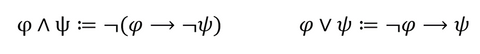When I started my undergraduate degree in maths, one of the first things I learned is that maths is a language. Well, several languages in fact. Each subject area has its own set of axioms and notation, and gaining understanding and knowledge in any given area requires patience and to slowly learn that language. Unfortunately, the time needed to break down the different symbols and definitions and really get to grips with a new concept is not something that many people have outside of the university. In order to continue sharing our beautiful equations with a wider audience, here’s a guide to some more of our favourite formula!In a first-order logic language it is possible to define the logical conjunction (‘and’) and disjunction (‘or’) using the symbols for logical negation and implication.

First-order logic provides a language from which all mathematical theories can be formulated. The alphabet of this language is made of 8 categories of symbols including the ‘negation’ and implication symbol used above but crucially, to save time when completing logical proofs, not the ‘and’ and ‘or’. This neat definition allows them to still be used freely, again saving mathematicians a lot of time!

The first study of modern logic is credited to George Boole, an English mathematician well known for his contributions to differential equations and algebraic logic such as Boolean Algebra, which he introduced in his first book The Mathematical Analysis of Logic in 1847. The conventions and language used has transitioned over the years as mathematicians searched for greater consistency across different areas. It was not until the end of the 20th century that first-order logic became standard for research in the foundations of mathematics.The determinant of a matrix 𝐴, denoted ′det(𝐴)′ above, with n rows and n columns can be calculated using this equation, the Leibniz formula

A matrix is a rectangular array of numbers arranged in rows and columns; they have applications in almost every field of science. Square matrices, where the number of rows and columns are the same such as the ‘n x n’ matrix A above, are commonly used in Linear Algebra – for example, to represent linear transformations such as a rotation or reflection. Each matrix has a determinant, a number that will indicate certain properties of the matrix, and this determinant features heavily in methods for finding a matrix’s inverse and in solving systems of linear equations. The Leibniz formula simplifies the task of calculating this value, saving mathematics a lot of time!

The use of the group theory and the symmetric group Sn whose elements represent permutations, is particularly neat and satisfying in this equation; however, alternative formula for the determinant can be constructed using concepts from other from areas of mathematics.

This formula is named in honour of George Leibniz, a German mathematician and philosopher who studied in the 17th century, a period often referred to as the ‘Age of Enlightenment’.If u and v are vectors defined over an inner product space (a vector space combined with a structure called an ‘inner product’ that satisfies certain conditions), then the above Cauchy-Schwarz inequality is true.

This is a general statement for a very widely used tool in mathematics, perhaps easier to visualise in the context of the inner product space of the real numbers in the nth dimension where the inner product is defined as the more commonly known ‘dot product’. There are several equations in varying areas of mathematics that follow as a direct consequence of Cauchy-Schwarz, such as the triangle inequality in analysis, determining the angle between two vectors in geometry and the covariance inequality in probability theory.

The inequality for sums was first published by Augustin-Louis Cauchy in 1821 and later for integrals by Hermann Schwarz in 1888, hence ‘Cauchy-Schwarz’. Cauchy was a French mathematician, engineer, and physicist. A prolific writer, he wrote approximately 800 research articles and five complete textbooks on a variety of topics and consequently his name appears before more theorems and concepts than perhaps any other mathematician. The lesser-known German mathematician Schwarz originally studied chemistry; however, he was convinced to change to mathematics by his father-in-law, the famous mathematician Ernst Eduard Kummer.

- Lucy Chats Maths